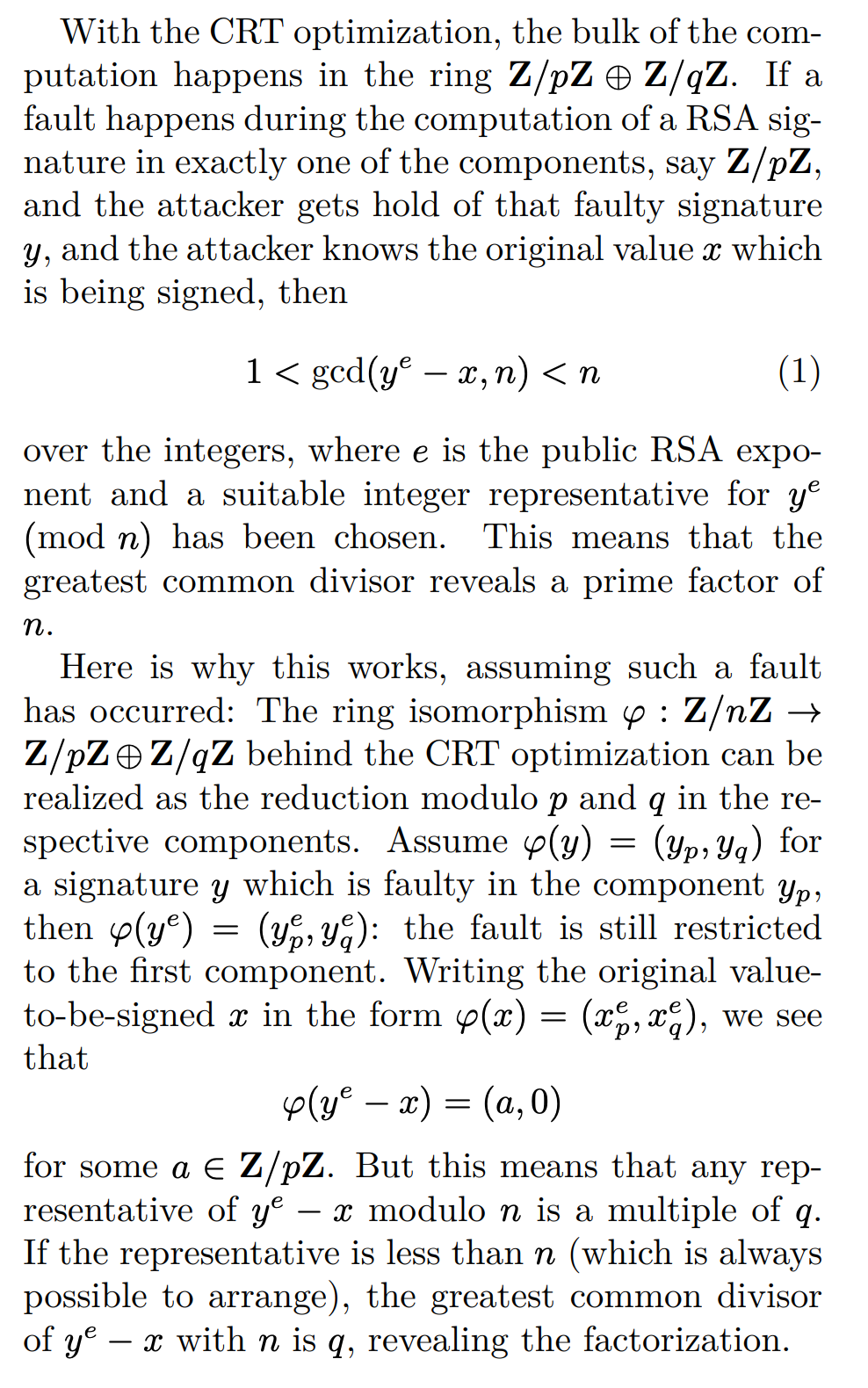# Two Easy Ways to Factor RSA

## Introduction

https://id0-rsa.pub/problem/15/

https://id0-rsa.pub/problem/45/

## Factor n with fault attack

### RSA-CRT optimization

paper：https://people.redhat.com/~fweimer/rsa-crt-leaks.pdf

• $\bmod{q}$：显然，$m \equiv m_q\pmod{q}$

• $\bmod{p}$：代入一下$h, q_{inv}$，$m\equiv m_q + q^{-1} \cdot (m_p - m_q) \cdot q \equiv m_o \pmod{p}$

### Arjen Lenstra’s CRT attack1. 某些任意精度的整数库出问题了。
2. 在读写数据时有条件竞争
3. CPU的运算单元出问题了（CPU本身问题或者外部环境影响）。
4. 私钥$(p, q, d_p, d_q, q_{inv})$中某部分变了。
5. CPU缓存或者内存受影响导致bit errors。

## Factor n given public exponent && private exponent1 2 3 4 5 6 7 8 9 10 11 12 13 14 15 16 17 18 19 20 21 22 23 24 25 26 27 28 29 30 31 32 33 34 35 36 37 38 39 40 41 42 43 44 45 46 47 48 49 50 51 52 53 54 55 56 57  In : from Crypto.Util.number import * In : p = getPrime(50) In : q = getPrime(50) In : n = p*q In : e = 0x10001 In : d = inverse(e, (p-1)*(q-1)) In : p, q, n, e, d Out: (595736391353269, 1115779042489183, 664710180320111683519664189227, 65537, 537877996898485924520932355009) In : kphi = e*d - 1 In : kphi Out: 35250910282736072035328343750224832 In : pow(2, kphi, n) Out: 1 In : pow(2, kphi // 2, n) Out: 1 In : pow(2, kphi // 4, n) Out: 1 In : pow(2, kphi // 8, n) Out: 1 In : pow(2, kphi // 16, n) Out: 1 In : pow(2, kphi // 32, n) Out: 78760480679820634213819949631 In : pow(2, kphi // 16, p) Out: 1 In : pow(2, kphi // 16, q) Out: 1 In : pow(2, kphi // 32, q) Out: 1 In : pow(2, kphi // 32, p) Out: 595736391353268 In : p - 1 Out: 595736391353268 

NCTF 2019 babyRSA

Load Comments?